### Surface Values vs. Underlying Detail

Calculations in a Client Table—especially those that work at a subtotal or total level are handled differently than many would expect. The issue has to do with the concept of calculations based on surface values vs. the underlying data that creates the surface values.

By default, break level summary fields simply add up the column values at the surface level and display a subtotal or total for the column or row. The individual column values are summary values of detail level data. More importantly they represent the lowest level of detail based on the fields in the view.

### An Example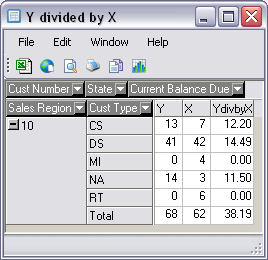In this simple table we see counts in the first two columns (Y and X) followed by a derived column that divides Y by X. Notice the subtotal level for Region 10—the value 38.19 is not the result of a calculation that divides 68 by 62, which we would expect. This value is just a subtotal of all the values in the column (12.20, 14.49, 0.00, 11.50, and 0.00)—just like the subtotals for columns X and Y. Here is another issue. In the row for the Cust Type ‘NA’, the value of 11.50 is not the result of 14 divided by 3. The correct value is 4.67.

The issue here is the underlying detail. The values for NA are really a row-level subtotal, and all subtotals are the result of the column values at the lowest available detail in the view.

We will use a technique that uses the surface values to calculate Y/X. This technique involves a change to the fields specified in the table as Rows(regon and ctype) as well as the lowest level detail field for this view—Cusno.

To change your summary calculations for the subtotal breaks, start in the Client Table design screen, and select Format\Group Footer Editor on the menu. Then perform the following three steps:

1. In the Define Summary Values panel, select a Row field. Again, you must select all row fields in the table as well as the field that represents the lowest level of detail (see last section).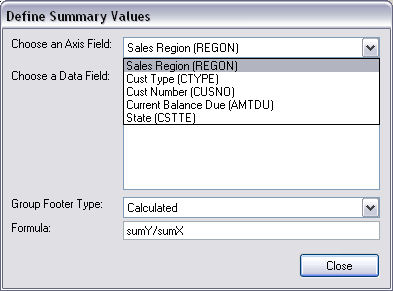2. Select a summary calculation.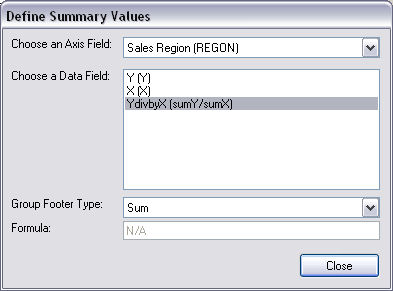3. Select ‘Calculated’ from the Group Footer Type list.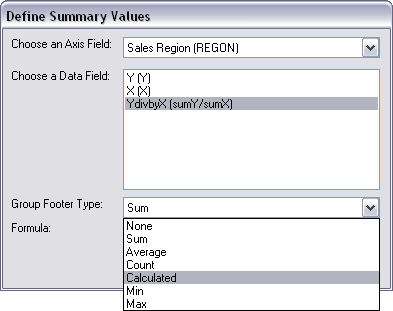After step 3 above, the Formula value will automatically fill in with the selected calculation.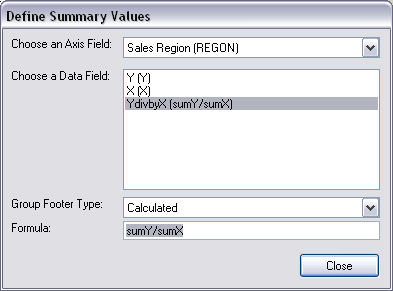When finished with all Row field/summary calculation combinations, press the Close button.

The final result looks like: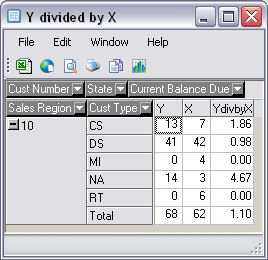### Lowest Detail Level Field

The low-level detail field will always be the first field displayed (left most) above the Row Fields of the table. (Cust Number in this example)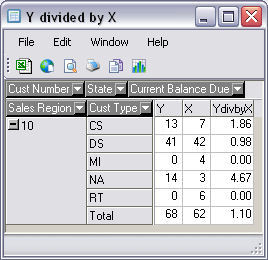Still have questions? We can help. Submit a case to Technical Support.